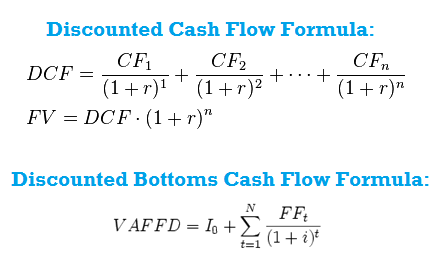# What is Discounted Cash Flow (DCF)? Definition, Analysis, Examples, Problems5
(6)

### Discounted Cash Flow Definition:

In Finance, the method of discounted cash flow, discounted cash flow or discounted bottoms cash flow (DCF for its acronym) is used to evaluate a project or an entire company. DCF methods determine the present value of future cash flows discounting them at a rate that reflects the cost of capital contributed. This is necessary because the cash flows in different periods cannot be compared directly because it is not the same to have a lot of money now than in the future.

DCF (Discounted Cash Flow) Procedure Involves Two Major Problems:

• The prognosis of projected cash flows.
• Determining the cost of capital appropriate.

The analysis of cash flow is widely used in corporate finance for asset valuations or valuation of investment projects. The result of the assessment is highly susceptible to the estimates made ​​for the preparation of these two variables.

###Discounted Cash Flow Calculation:

Detail description of discounted cash flow formula as: ‘DPV‘ as (discounted present value) of future cash flow (FV). ‘FV‘ describe the nominal future period value of a cash flow balance. ‘r‘ defines the discount rate or interest rate. ‘n‘ is the number of time in years before the occurrence of future cash flow.

### Discounted Bottoms Cash Flow Calculation:

This calculation system is using the technique of Net Present Value, calculate the value on the valuation date of all cash flows that will occur in the future and will allow us also to value a company to calculate the value of a new investment project, a financial asset.

Detailed description of discounted bottoms cash flow formula as: ‘VAFFD‘ is the discounted present value of future cash flows (FF). ‘I0‘ is the initial investment to launch the project. ‘FF‘ is the nominal value of cash flows in a given future period. ‘I‘ is the discount rate which is the opportunity cost of funds invested, considering the risk factor. ‘N‘ is the number of periods discounted.

The valuation is performed is always based on three factors:

• Funds.
• Location in time.
• Risk.

The question to answer is: how much is expected to be worth the expected cash flows after the firm has made ​​the investments? The answer is shaped through the method called discounted cash flow.

###Discounted Cash Flow Example:

Here in this image you can see the sample template of discounted cash flow methodology. There are various different ways to calculate or evaluate the valuation of the company or the business. Discounted cash flow reflect the value of the business towards the sum of future projected cash flow. This will assist you in taking the decision whether to sell or buy the stock of the company.

### Discounted Cash Flow Valuation of a Company:

Then the net present value of an investment is the present value of all cash flows and future, discounted at the opportunity cost of these cash flows. If instead of analyzing an individual investment, which we are evaluating is the present value of a going concern, the net present value is the present value of all cash flows expected the company to produce all his life: this is the value of a company, and in the case of listed companies, is reflected in the total value of all its shares.

The analysis through f luxuries discounted cash considers the business as a series of risky cash flows that occur in the future. The analyst’s task is first to predict what the future expected cash flows will be, period by period, and second, discounting the projected values ​​to the current time, the opportunity cost of those funds. The opportunity cost is the return that a company could expect to win in an alternative investment involving the same risk.

The opportunity costs consist partly of the time value of money (the return of a risk – free investment). This is the return that can be obtained only by being patient, without any risk. The opportunity cost also includes a price risk (the extra income that can be expected in relation to risk one is willing to endure).

In some cases, instead of determining the net present value, which is an absolute measure of performance because it indicates the largest value of a company by the fact that he faced a particular investment, working with a relative measure of performance, which is the internal rate of return.

The internal rate of return is known as the decreased rate that equates the present value of the income and expenses of an investment fund. It is the return on an investment, measured as a percentage of the amount invested.

Returning to the current value, it can be seen that the final value obtained, depends on two values:

• The value assigned to the expected funds.

If this value increases, it increases the current value of the company; if low, low also the current value.

• The discount rate used.

If the rate increases; the low current value, and if the rate low, the current value increases. As the value of the rate is closely related to the investment risk, it can be seen that the riskier it is assumed that investment, the lower its current value.

Read E-Learning Tutorial Courses - 100% Free for All

Click on a star to rate it!

Average rating 5 / 5. Vote count: 6

No votes so far! Be the first to rate this post.

We are sorry that this post was not useful for you!

Let us improve this post!

Tell us how we can improve this post?

### 2 thoughts on “What is Discounted Cash Flow (DCF)? Definition, Analysis, Examples, Problems”

1.Really nice information about Discounted Cash Flow (DCF). Thanks for sharing it!

2.I do not even know how I ended up here, but I liked your discounted cash flow example problem statement. Thanks a Lot!